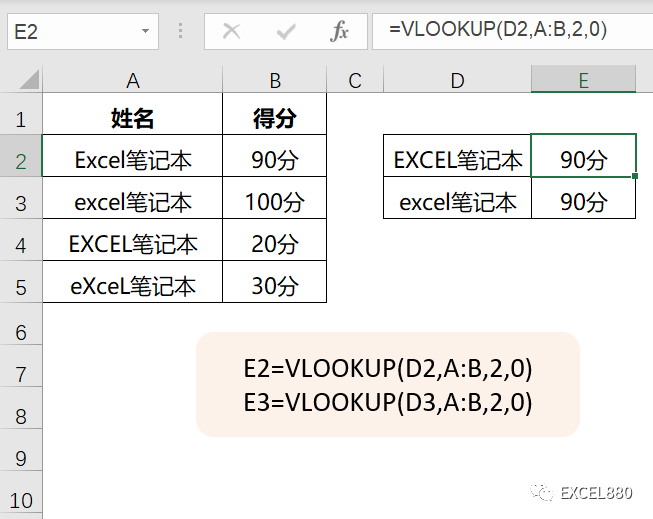# VLOOKUP函数出错的10种原因分析 Excel/wps函数

312 次浏览

VLOOKUP出错？不要抓狂，参数挨个过一遍，无非就这几种问题。

1.第一参数和查询区域是否格式相同？

=VLOOKUP(D3,A:B,2,0)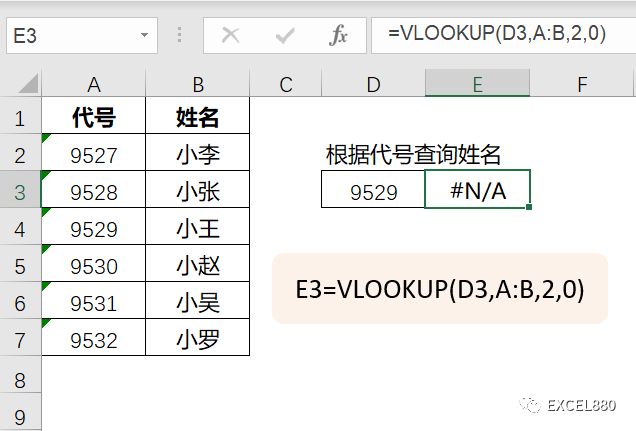=VLOOKUP(D3&””,A:B,2,0)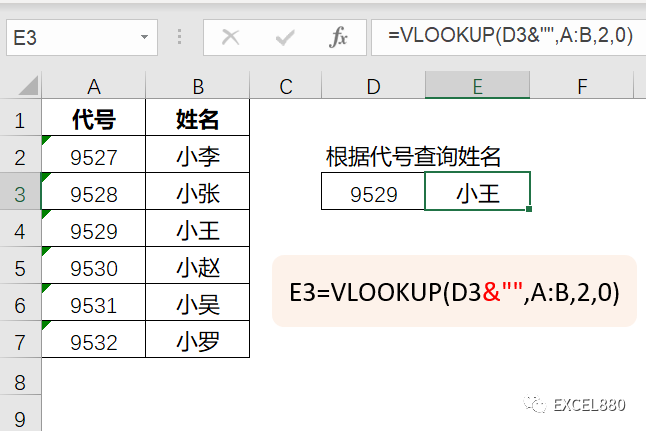2. 第二参数是否锁定？

=VLOOKUP($F2,$A$2:$D\$10,COLUMN(B:B),FALSE)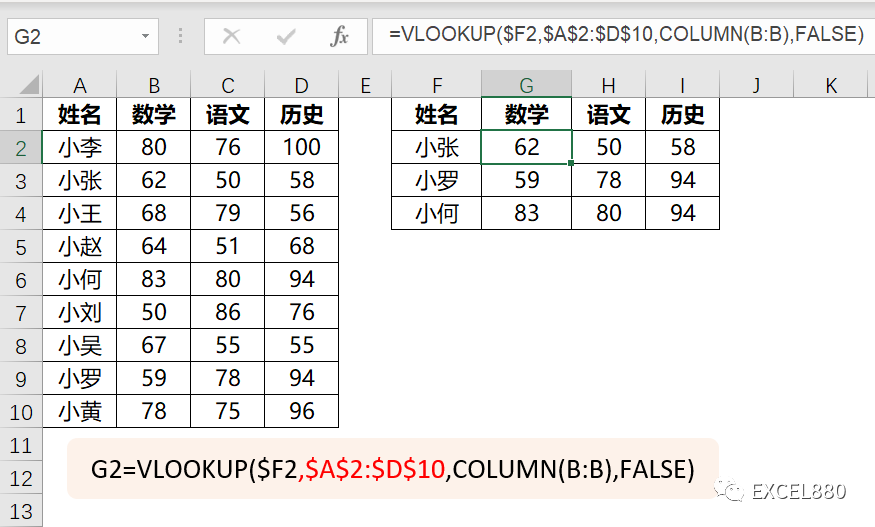3．第二参数范围是否正确？

=VLOOKUP(“小王”,A:E,4,FALSE)

“小王“所在的B列没有被置于第二参数A:E的首列，返回错误。

=VLOOKUP(“小王”,B:E,3,FALSE)

“小王“所在的B列被置于第二参数B:E的首列，正确。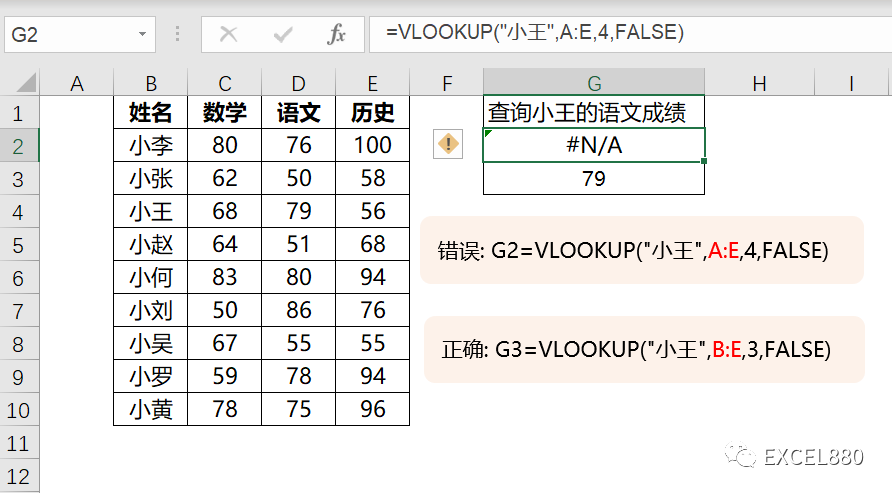4. 第四参数是否从首列开始数？

=VLOOKUP(“小王”,C1:K9,9,0)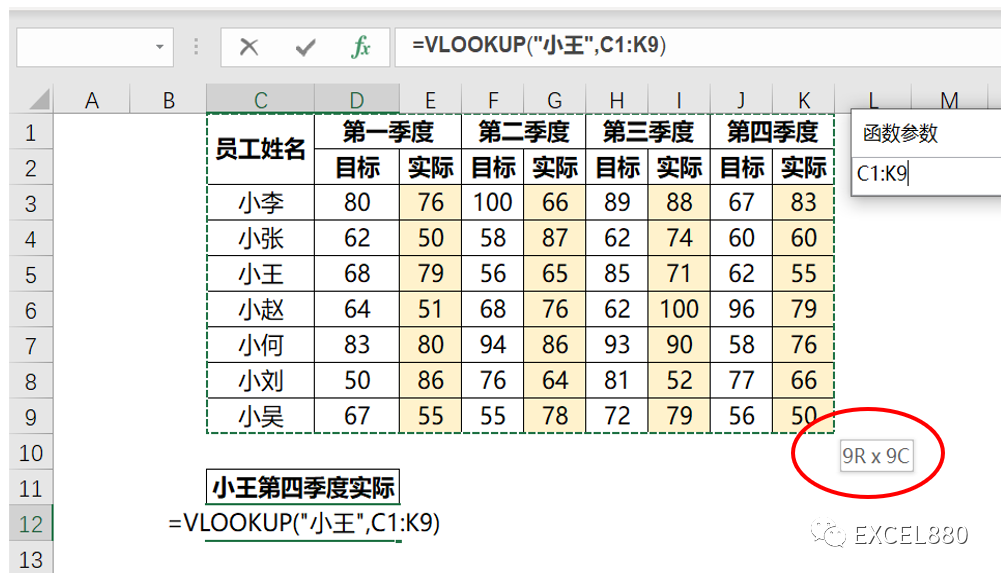5.第三参数是不是负数？

VLOOKUP不能从右往左查询，第三参数只能是正整数。

=VLOOKUP(D4,A:B,-1,0)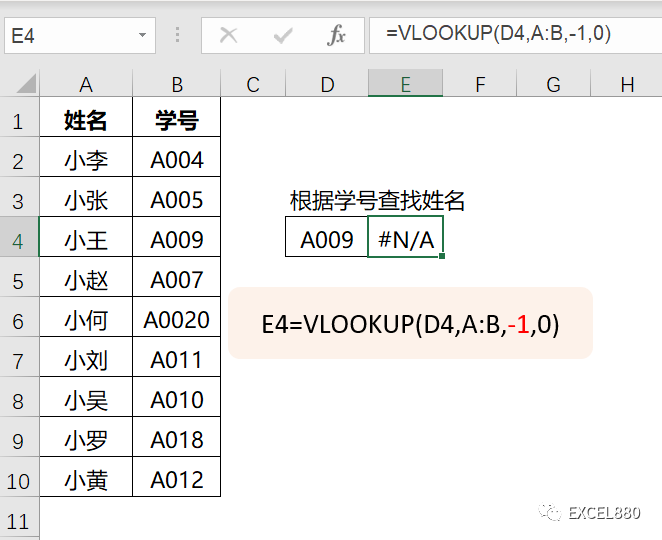6.省略第四参数？

=VLOOKUP(D4,A:B,2)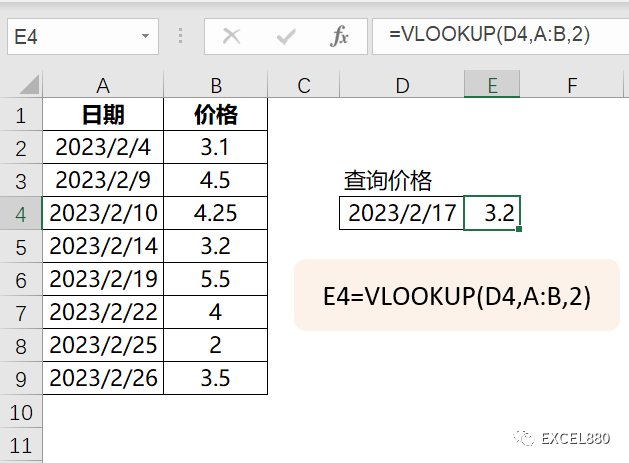7.公式完成后是否有改变数据结构

=VLOOKUP(F2,A:D,4,0)

=VLOOKUP(F2,A:D,4,0)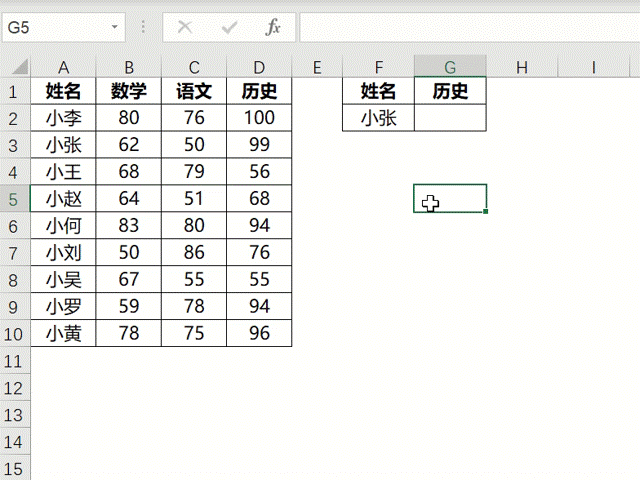8.大小写？

VLOOKUP:我天天这么忙，还要给你们区分大小写？

=VLOOKUP(D2,A:B,2,0)

=VLOOKUP(D3,A:B,2,0)